Courses

# Rectifiers & Filters Electrical Engineering (EE) Notes | EduRev

## Electronic Devices

Created by: Cstoppers Instructors

## Electrical Engineering (EE) : Rectifiers & Filters Electrical Engineering (EE) Notes | EduRev

The document Rectifiers & Filters Electrical Engineering (EE) Notes | EduRev is a part of the Electrical Engineering (EE) Course Electronic Devices.
All you need of Electrical Engineering (EE) at this link: Electrical Engineering (EE)

INTRODUCTION

For the operation of most of the electronics devices and circuits, a d.c. source is required. So it is advantageous to convert domestic a.c. supply into d.c.voltages. The process of converting a.c. voltage into d.c. voltage is called rectification. This is achieved with i) Step-down Transformer, ii) Rectifier, iii) Filter and iv) Voltage regulator circuits.

These elements constitute d.c. regulated power supply shown in the fig 1 below.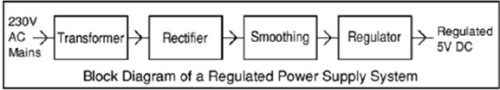Fig. 1

• Transformer – steps down 230V AC mains to low voltage AC.
• Rectifier – converts AC to DC, but the DC output is varying.
• Smoothing – smooth the DC output from varying greatly to a small ripple.
• Regulator – eliminates ripple by setting DC output to a fixed voltage.

The block diagram of a regulated D.C. power supply consists of step-down transformer, rectifier, filter, voltage regulator and load. An ideal regulated power supply is an electronic circuit designed to provide a predetermined d.c. voltage Vo which is independent of the load current and variations in the input voltage and temperature. If the output of a regulator circuit is a AC voltage then it is termed as voltage stabilizer, whereas if the output is a DC voltage then it is termed as voltage regulator.

RECTIFIER:

Any electrical device which offers a low resistance to the current in one direction but a high resistance to the current in the opposite direction is called rectifier. Such a device is capable of converting a sinusoidal input waveform, whose average value is zero, into a unidirectional waveform, with a non-zero average component. A rectifier is a device, which converts a.c. voltage (bi-directional) to pulsating d.c. voltage (unidirectional).

Characteristics of a Rectifier Circuit:

Any electrical device which offers a low resistance to the current in one direction but a high resistance to the current in the opposite direction is called rectifier. Such a device is capable of converting a sinusoidal input waveform, whose average value is zero, into a unidirectional waveform, with a non-zero average component.

A rectifier is a device, which converts a.c. voltage (bi-directional) to pulsating d.c..

Load currents: There are two types of output current i.e. average or d.c. current and RMS currents.

Average or DC current: The average current of a periodic function is defined as the area of one cycle of the curve divided by the base.

It is expressed mathematically as
1) Average value/dc value/mean value=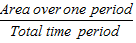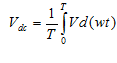ii)  Effective (or) R.M.S current:

The effective (or) R.M.S. current squared of a periodic function of time is given by the area of one cycle of the curve, which represents the square of the function divided by the base.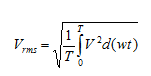iii) Peak factor:

It is the ratio of peak value to R.M.S value.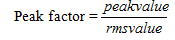iv) Form factor:

It is the ratio of R.M.S value to average value.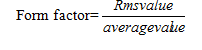v) Ripple Factor : It is defined as ration of R.M.S. value of a.c. component to the d.c. component in the output is known as “Ripple Factor”.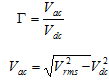vi)  Efficiency (η):

It is the ratio of d.c output power to the a.c. input power. It signifies, how efficiently the rectifier circuit converts a.c. power into d.c. power.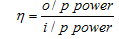vii) Peak Inverse Voltage (PIV):

It is defined as the maximum reverse voltage that a diode can withstand without destroying  the junction.

viii) Transformer Utilization Factor (UTF):

The d.c. power to be delivered to the load in a rectifier circuit decides the rating of the

transformer used in the circuit. So, transformer utilization factor is defined as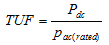ix) % Regulation:

The variation of the d.c. output voltage as a function of d.c. load current is called regulation. The percentage regulation is defined as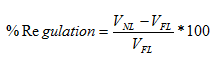For an ideal power supply, % Regulation is zero.

Offer running on EduRev: Apply code STAYHOME200 to get INR 200 off on our premium plan EduRev Infinity!

,

,

,

,

,

,

,

,

,

,

,

,

,

,

,

,

,

,

,

,

,

;